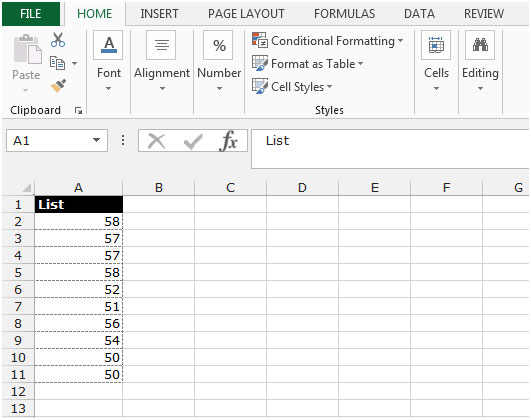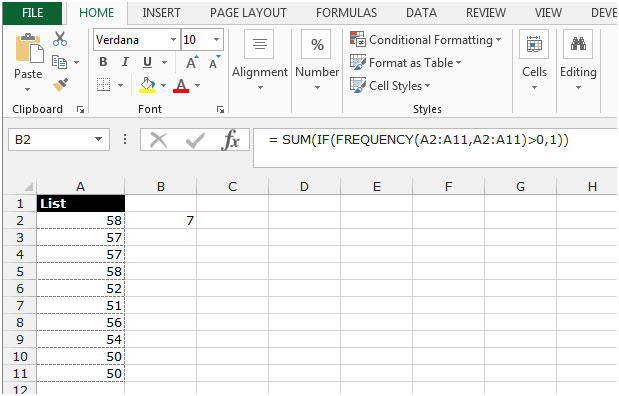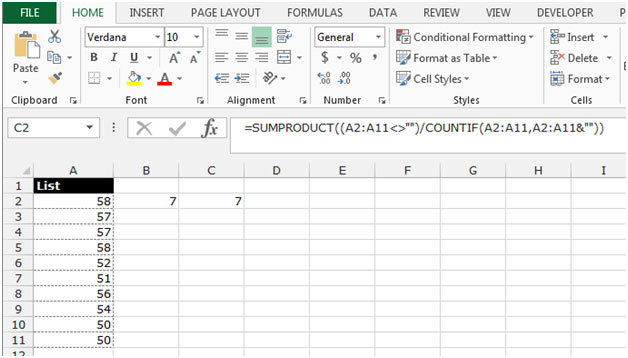# Counting Unique Numeric Values or Unique Data in a List in Microsoft Excel

In this article, we will learn how to count the unique numeric values or unique data in a list. We can use two types of combinations of formulas in Microsoft Excel to get the distinct count.

Let’s take an example to understand how we can count the distinct numeric values or unique list.

We have a number list in range A2:A11.To count the unique values in a range, follow below given steps:-

• Write the formula in cell B2.
• = SUM(IF(FREQUENCY(A2:A11,A2:A11)>0,1))
• Press Enter on your keyboard.
• The function will return 7. It means 7 numbers are unique out of 10 numbers.Another formula we can use for the same result.

Use this mentioned formula =SUMPRODUCT((A2:A11<>"")/COUNTIF(A2:A11,A2:A11&""))in the cell C2. You will get the same result.This is the way we can count the unique numeric values in a list or range in Microsoft Excel.We would love to hear from you, do let us know how we can improve, complement or innovate our work and make it better for you. Write us at info@exceltip.com

## Users are saying about us...

1.Worked great. I used the second function.

2.Can you share with me how to write an Excel formula to count from both cells which have combination of text and numeric in the same cell. Example;

B2 is 3N
C2 is M4

So how to count or add these 2 cells to become 7

Your kind assist is greatly appreciate and grateful.

tqvm

Terms and Conditions of use

The applications/code on this site are distributed as is and without warranties or liability. In no event shall the owner of the copyrights, or the authors of the applications/code be liable for any loss of profit, any problems or any damage resulting from the use or evaluation of the applications/code.